# Computer Networks and Internets 5 e By Douglas

• Slides: 46Computer Networks and Internets, 5 e By Douglas E. Comer Lecture Power. Points Adapted from the notes By Lami Kaya, [email protected] org © 2009 Pearson Education Inc. , Upper Saddle River, NJ. All rights reserved. 1Chapter 11 Multiplexing and Demultiplexing (Channelization) 2Topics Covered • • • • 11. 1 Introduction 11. 2 The Concept of Multiplexing 11. 3 The Basic Types of Multiplexing 11. 4 Frequency Division Multiplexing (FDM) 11. 5 Using a Range of Frequencies Per Channel 11. 6 Hierarchical FDM 11. 7 Wavelength Division Multiplexing (WDM) 11. 8 Time Division Multiplexing (TDM) 11. 9 Synchronous TDM 11. 10 Framing Used in the Telephone System Version of TDM 11. 11 Hierarchical TDM 11. 12 The Problem with Synchronous TDM: Unfilled Slots 11. 13 Statistical TDM 11. 14 Inverse Multiplexing 11. 15 Code Division Multiplexing 3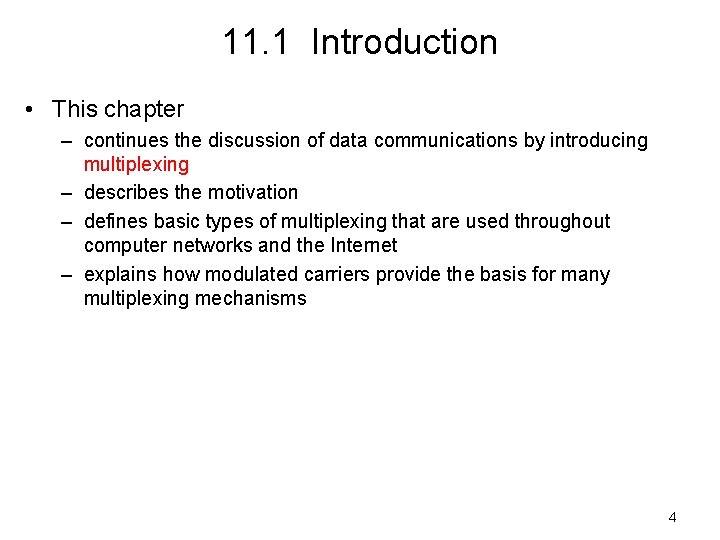11. 1 Introduction • This chapter – continues the discussion of data communications by introducing multiplexing – describes the motivation – defines basic types of multiplexing that are used throughout computer networks and the Internet – explains how modulated carriers provide the basis for many multiplexing mechanisms 4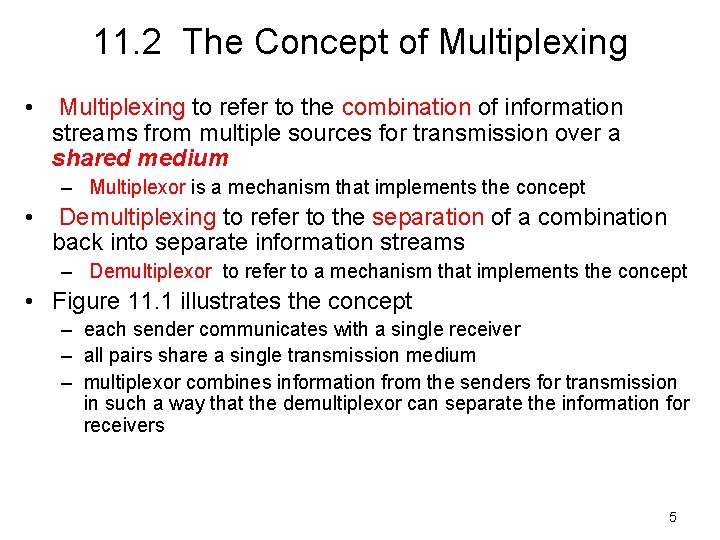11. 2 The Concept of Multiplexing • Multiplexing to refer to the combination of information streams from multiple sources for transmission over a shared medium – Multiplexor is a mechanism that implements the concept • Demultiplexing to refer to the separation of a combination back into separate information streams – Demultiplexor to refer to a mechanism that implements the concept • Figure 11. 1 illustrates the concept – each sender communicates with a single receiver – all pairs share a single transmission medium – multiplexor combines information from the senders for transmission in such a way that the demultiplexor can separate the information for receivers 5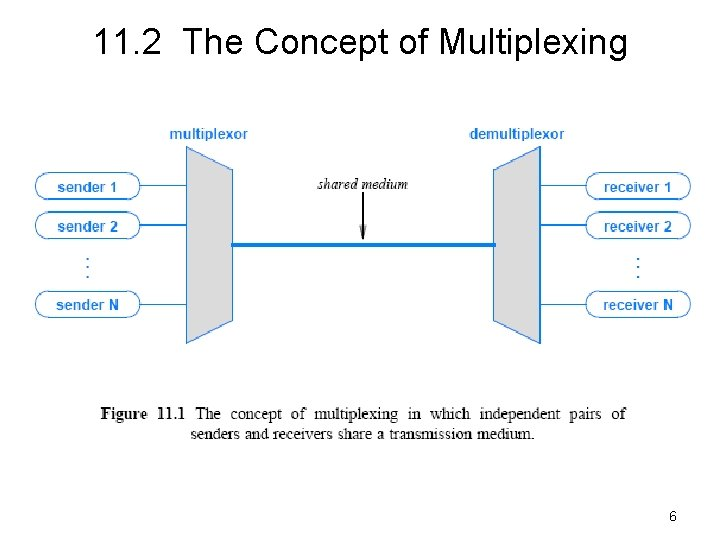11. 2 The Concept of Multiplexing 6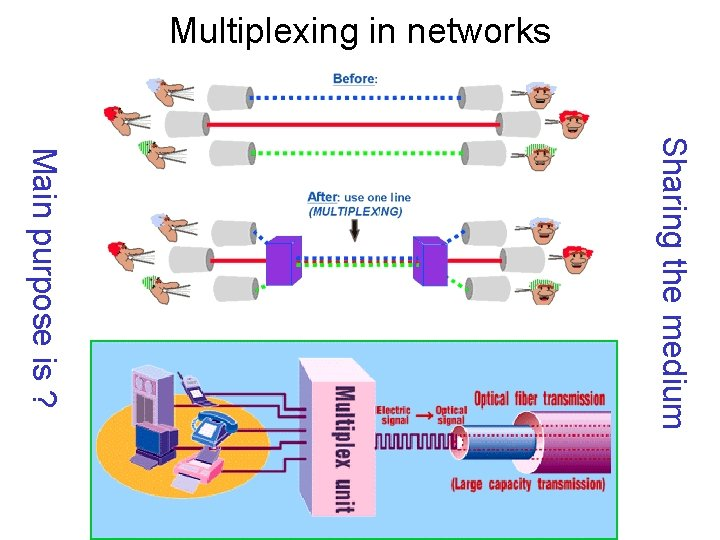Multiplexing in networks Sharing the medium Main purpose is ?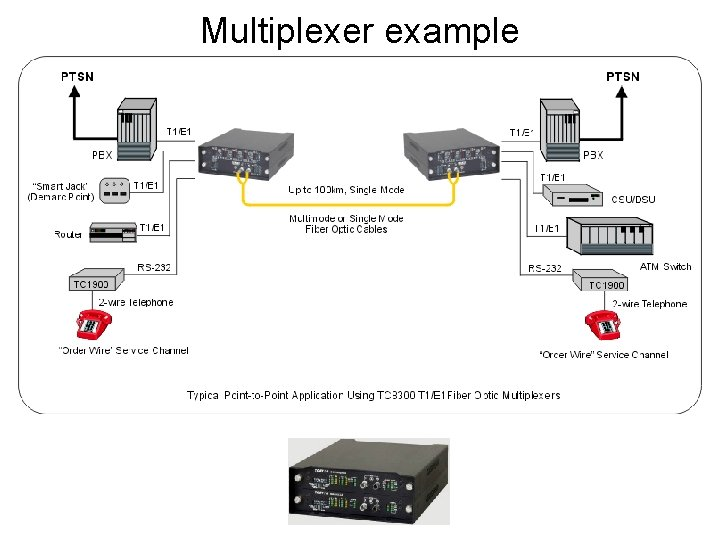Multiplexer example11. 3 The Basic Types of Multiplexing • There are four basic approaches to multiplexing that each have a set of variations and implementations – – Frequency Division Multiplexing (FDM) Wavelength Division Multiplexing (WDM) Time Division Multiplexing (TDM) Code Division Multiplexing (CDM) • TDM and FDM are widely used • WDM is a form of FDM used for optical fiber • CDM is a mathematical approach used in cell phone mechanisms 9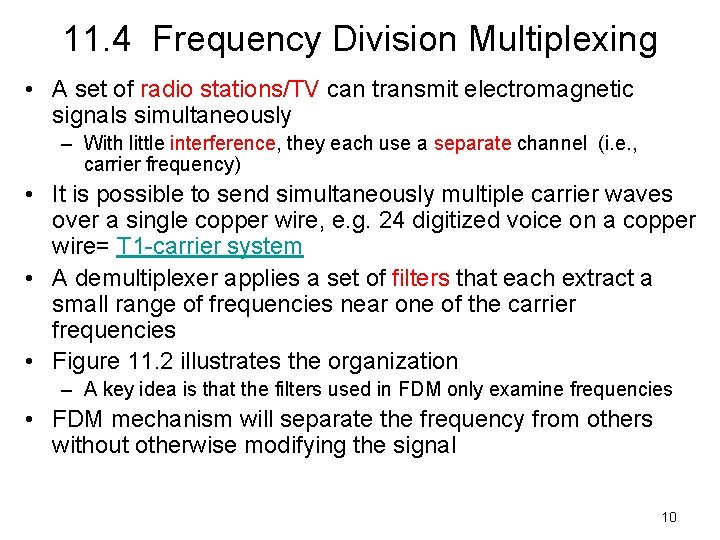11. 4 Frequency Division Multiplexing • A set of radio stations/TV can transmit electromagnetic signals simultaneously – With little interference, they each use a separate channel (i. e. , carrier frequency) • It is possible to send simultaneously multiple carrier waves over a single copper wire, e. g. 24 digitized voice on a copper wire= T 1 -carrier system • A demultiplexer applies a set of filters that each extract a small range of frequencies near one of the carrier frequencies • Figure 11. 2 illustrates the organization – A key idea is that the filters used in FDM only examine frequencies • FDM mechanism will separate the frequency from others without otherwise modifying the signal 1011. 4 Frequency Division Multiplexing 11Example usage of Multiplexing in DSSS (used in cell phone technology)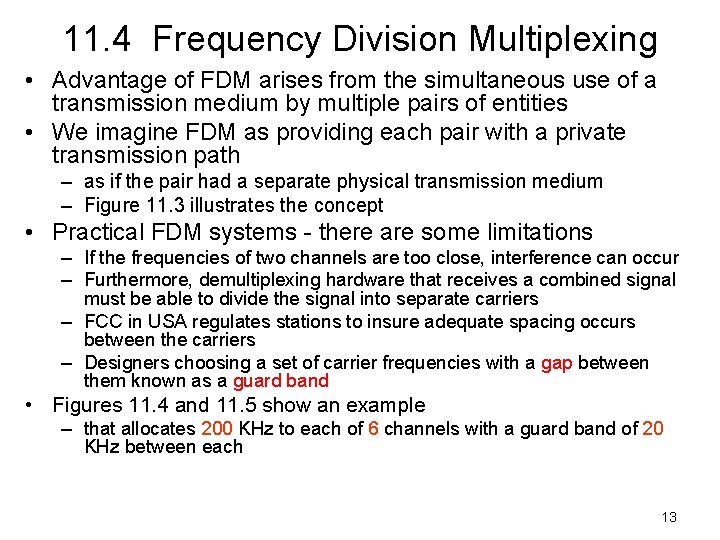11. 4 Frequency Division Multiplexing • Advantage of FDM arises from the simultaneous use of a transmission medium by multiple pairs of entities • We imagine FDM as providing each pair with a private transmission path – as if the pair had a separate physical transmission medium – Figure 11. 3 illustrates the concept • Practical FDM systems - there are some limitations – If the frequencies of two channels are too close, interference can occur – Furthermore, demultiplexing hardware that receives a combined signal must be able to divide the signal into separate carriers – FCC in USA regulates stations to insure adequate spacing occurs between the carriers – Designers choosing a set of carrier frequencies with a gap between them known as a guard band • Figures 11. 4 and 11. 5 show an example – that allocates 200 KHz to each of 6 channels with a guard band of 20 KHz between each 13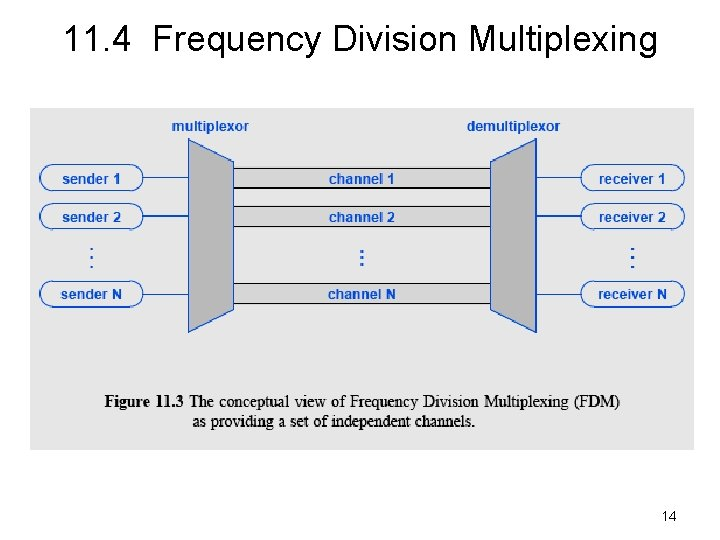11. 4 Frequency Division Multiplexing 14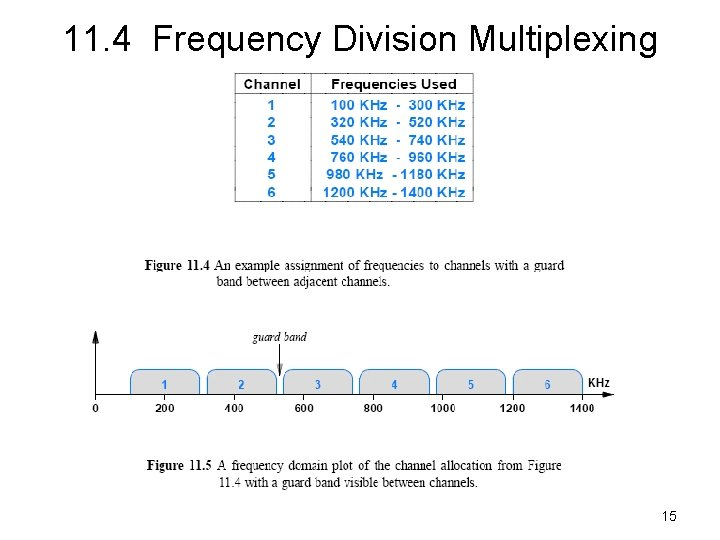11. 4 Frequency Division Multiplexing 15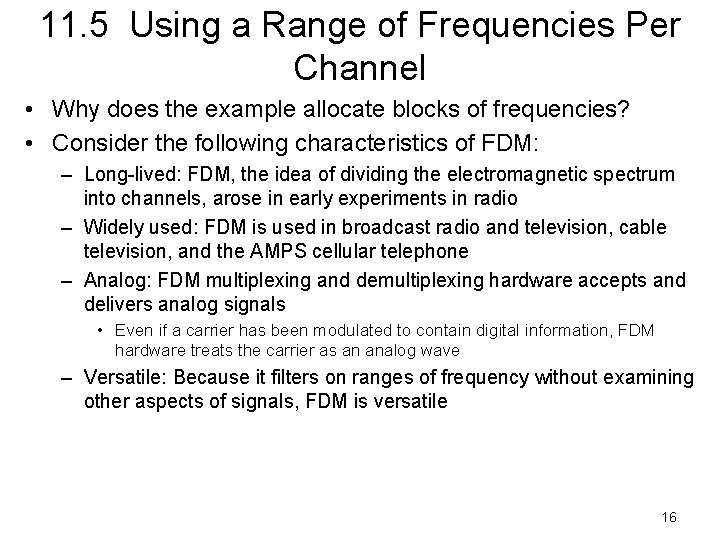11. 5 Using a Range of Frequencies Per Channel • Why does the example allocate blocks of frequencies? • Consider the following characteristics of FDM: – Long-lived: FDM, the idea of dividing the electromagnetic spectrum into channels, arose in early experiments in radio – Widely used: FDM is used in broadcast radio and television, cable television, and the AMPS cellular telephone – Analog: FDM multiplexing and demultiplexing hardware accepts and delivers analog signals • Even if a carrier has been modulated to contain digital information, FDM hardware treats the carrier as an analog wave – Versatile: Because it filters on ranges of frequency without examining other aspects of signals, FDM is versatile 1611. 5 Using a Range of Frequencies Per Channel • The analog characteristic has the disadvantage of making FDM susceptible to noise and distortion • Most FDM systems assign each sender and receiver pair a range of frequencies • FDM has the ability to choose how the frequencies can be used • There are two primary ways that systems use a range of frequencies – Increase the data rate – Increase immunity to interference • To increase the overall data rate – a sender divides the frequency range of the channel into K carriers – and sends 1/K of the data over each carrier 17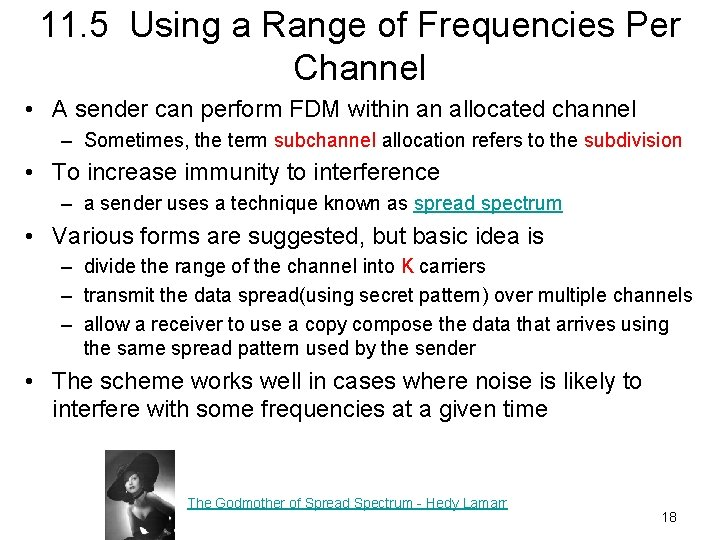11. 5 Using a Range of Frequencies Per Channel • A sender can perform FDM within an allocated channel – Sometimes, the term subchannel allocation refers to the subdivision • To increase immunity to interference – a sender uses a technique known as spread spectrum • Various forms are suggested, but basic idea is – divide the range of the channel into K carriers – transmit the data spread(using secret pattern) over multiple channels – allow a receiver to use a copy compose the data that arrives using the same spread pattern used by the sender • The scheme works well in cases where noise is likely to interfere with some frequencies at a given time The Godmother of Spread Spectrum - Hedy Lamarr 1811. 6 Hierarchical FDM • Flexibility in FDM arises from the ability of hardware to shift frequencies • If a set of incoming signals all use the frequency range between 0 and 4 KHz – multiplexing hardware can leave the first stage as is – map the second onto the range 4 KHz to 8 KHz – map the third onto the range 8 KHz to 12 KHz, and so on • Hierarchy in FDM multiplexors is that each maps its inputs to a larger, continuous band of frequencies • Figure 11. 6 illustrates the concept of hierarchical FDM 19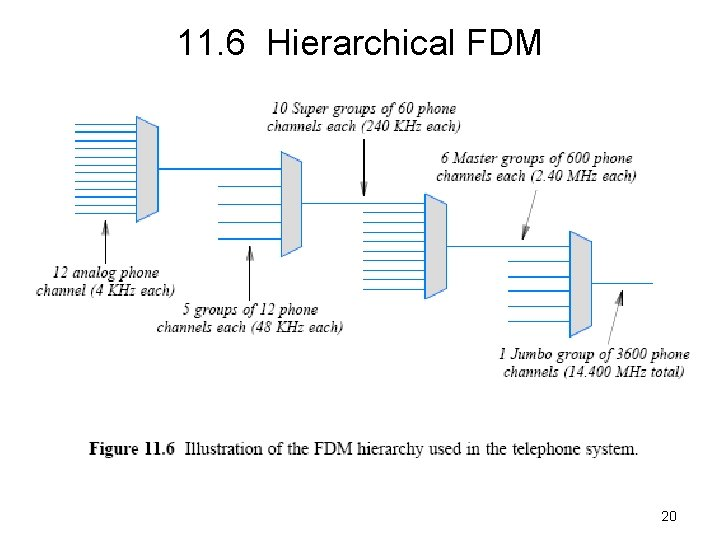11. 6 Hierarchical FDM 20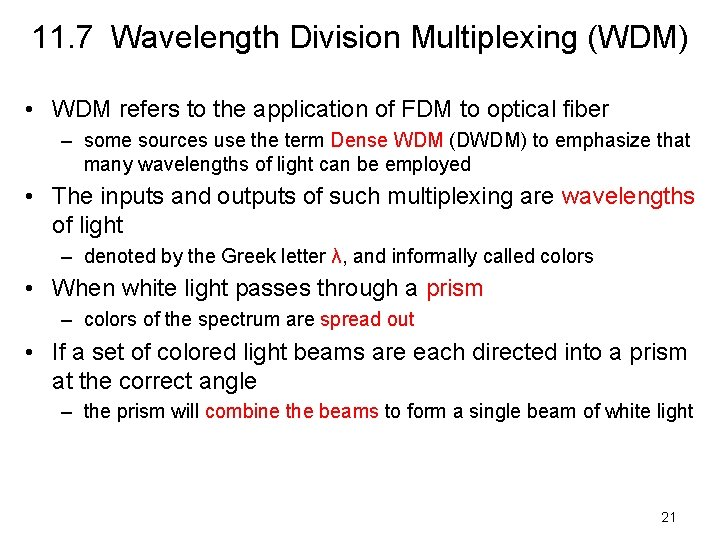11. 7 Wavelength Division Multiplexing (WDM) • WDM refers to the application of FDM to optical fiber – some sources use the term Dense WDM (DWDM) to emphasize that many wavelengths of light can be employed • The inputs and outputs of such multiplexing are wavelengths of light – denoted by the Greek letter λ, and informally called colors • When white light passes through a prism – colors of the spectrum are spread out • If a set of colored light beams are each directed into a prism at the correct angle – the prism will combine the beams to form a single beam of white light 21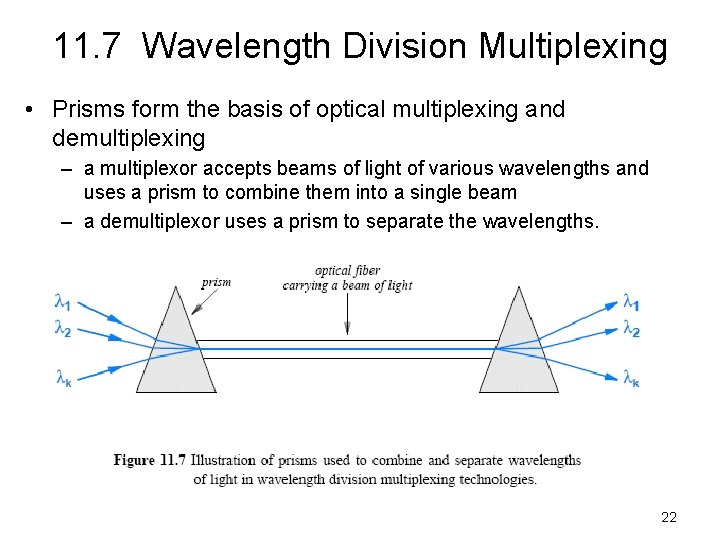11. 7 Wavelength Division Multiplexing • Prisms form the basis of optical multiplexing and demultiplexing – a multiplexor accepts beams of light of various wavelengths and uses a prism to combine them into a single beam – a demultiplexor uses a prism to separate the wavelengths. 22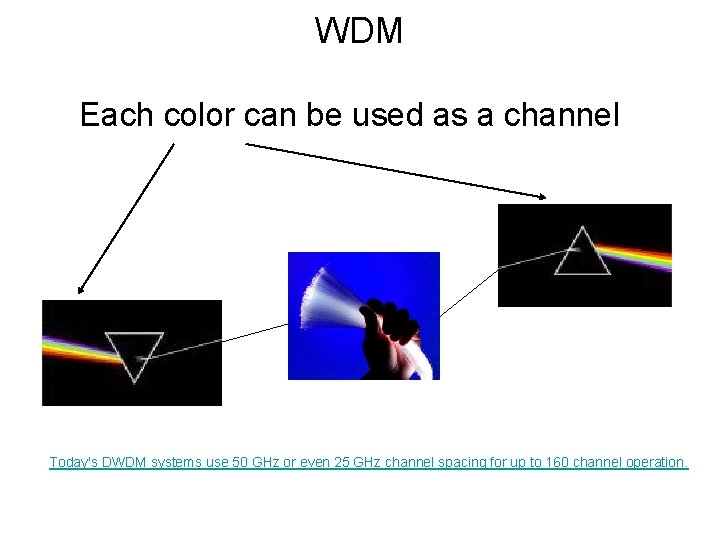WDM Each color can be used as a channel Today's DWDM systems use 50 GHz or even 25 GHz channel spacing for up to 160 channel operation.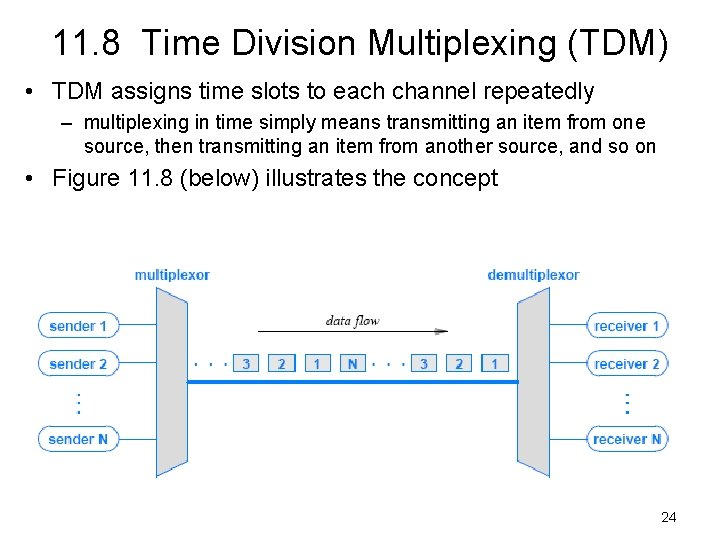11. 8 Time Division Multiplexing (TDM) • TDM assigns time slots to each channel repeatedly – multiplexing in time simply means transmitting an item from one source, then transmitting an item from another source, and so on • Figure 11. 8 (below) illustrates the concept 24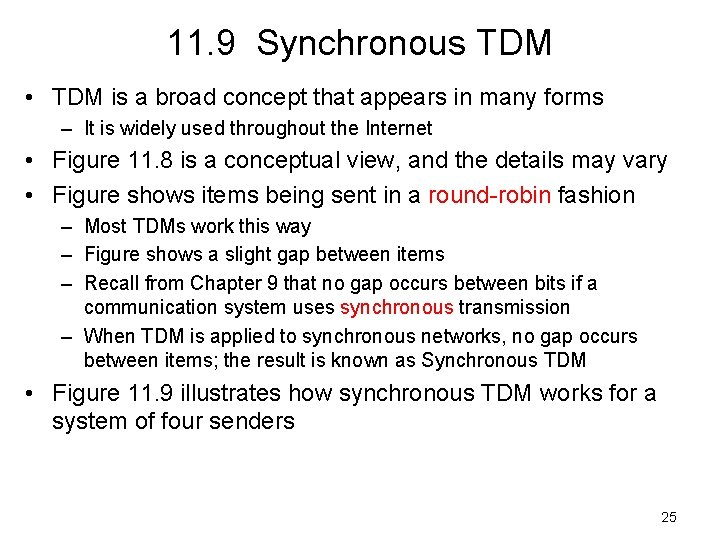11. 9 Synchronous TDM • TDM is a broad concept that appears in many forms – It is widely used throughout the Internet • Figure 11. 8 is a conceptual view, and the details may vary • Figure shows items being sent in a round-robin fashion – Most TDMs work this way – Figure shows a slight gap between items – Recall from Chapter 9 that no gap occurs between bits if a communication system uses synchronous transmission – When TDM is applied to synchronous networks, no gap occurs between items; the result is known as Synchronous TDM • Figure 11. 9 illustrates how synchronous TDM works for a system of four senders 2511. 9 Synchronous TDM 26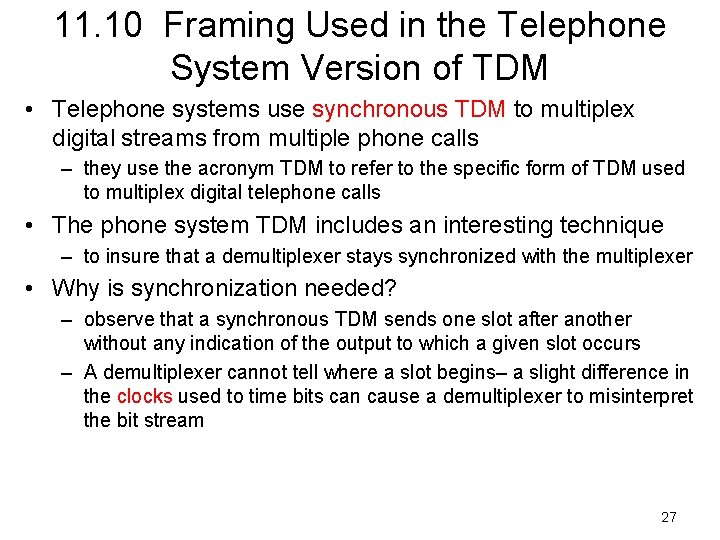11. 10 Framing Used in the Telephone System Version of TDM • Telephone systems use synchronous TDM to multiplex digital streams from multiple phone calls – they use the acronym TDM to refer to the specific form of TDM used to multiplex digital telephone calls • The phone system TDM includes an interesting technique – to insure that a demultiplexer stays synchronized with the multiplexer • Why is synchronization needed? – observe that a synchronous TDM sends one slot after another without any indication of the output to which a given slot occurs – A demultiplexer cannot tell where a slot begins– a slight difference in the clocks used to time bits can cause a demultiplexer to misinterpret the bit stream 27Up to here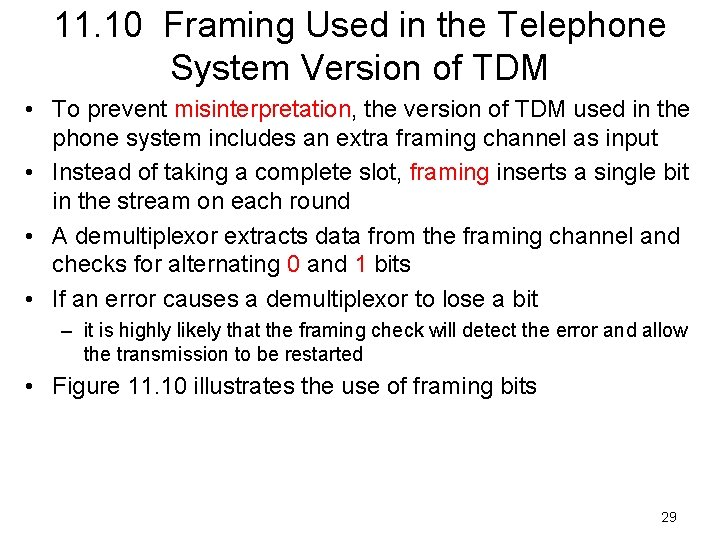11. 10 Framing Used in the Telephone System Version of TDM • To prevent misinterpretation, the version of TDM used in the phone system includes an extra framing channel as input • Instead of taking a complete slot, framing inserts a single bit in the stream on each round • A demultiplexor extracts data from the framing channel and checks for alternating 0 and 1 bits • If an error causes a demultiplexor to lose a bit – it is highly likely that the framing check will detect the error and allow the transmission to be restarted • Figure 11. 10 illustrates the use of framing bits 2911. 10 Framing Used in the Telephone System Version of TDM 3011. 11 Hierarchical TDM • Like FDM, TDM can be arranged in a hierarchy • The difference is that each successive stage of a TDM hierarchy uses N times the bit rate – In FDM, each successive stage uses N times the frequencies • Additional framing bits are added to the data – means that the bit rate of each successive layer of hierarchy is slightly greater than the aggregate voice traffic • Compare the example TDM hierarchy – in Figure 11. 11 with the FDM example in Figure 11. 6 3111. 11 Hierarchical TDM 32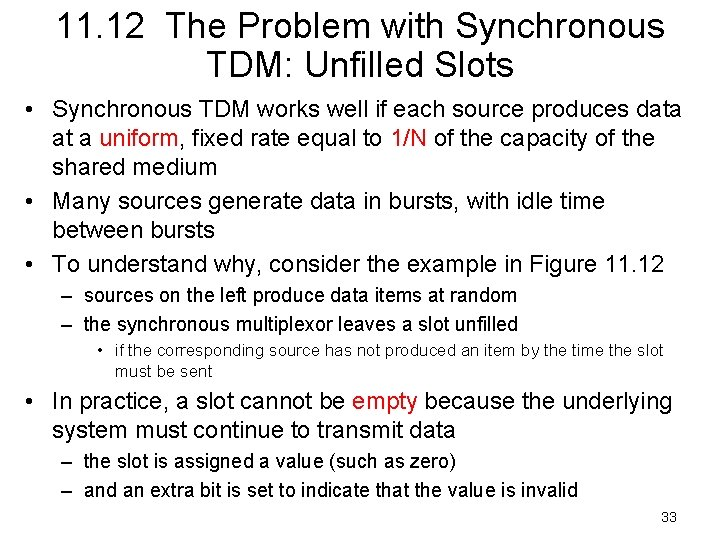11. 12 The Problem with Synchronous TDM: Unfilled Slots • Synchronous TDM works well if each source produces data at a uniform, fixed rate equal to 1/N of the capacity of the shared medium • Many sources generate data in bursts, with idle time between bursts • To understand why, consider the example in Figure 11. 12 – sources on the left produce data items at random – the synchronous multiplexor leaves a slot unfilled • if the corresponding source has not produced an item by the time the slot must be sent • In practice, a slot cannot be empty because the underlying system must continue to transmit data – the slot is assigned a value (such as zero) – and an extra bit is set to indicate that the value is invalid 33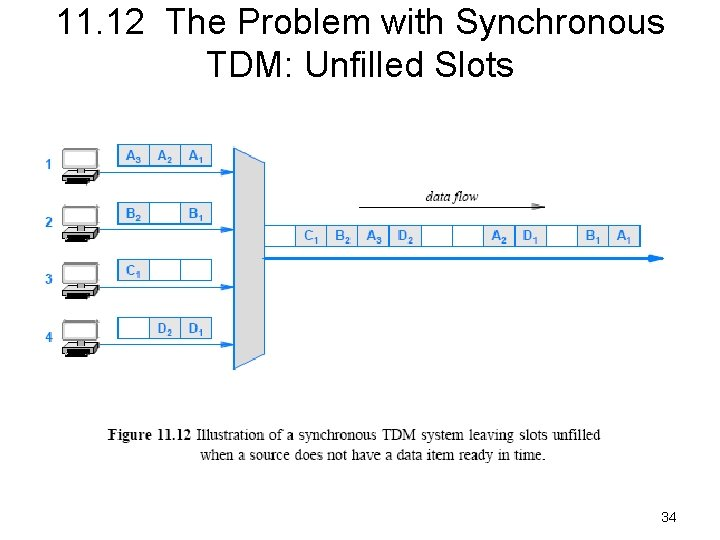11. 12 The Problem with Synchronous TDM: Unfilled Slots 34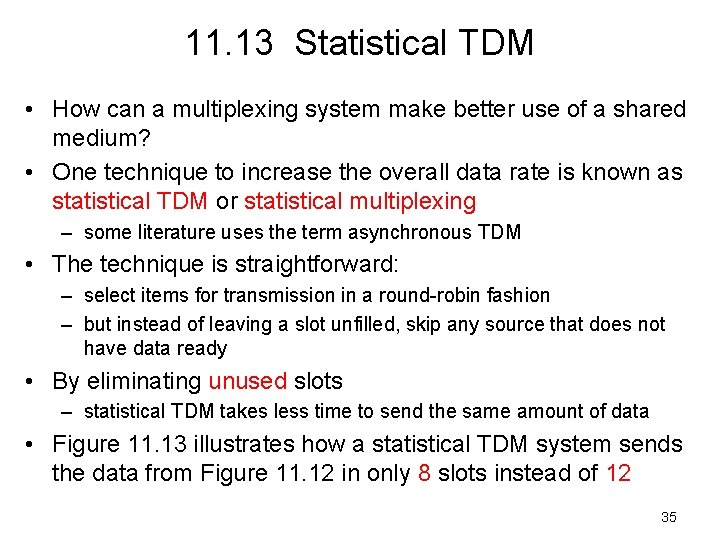11. 13 Statistical TDM • How can a multiplexing system make better use of a shared medium? • One technique to increase the overall data rate is known as statistical TDM or statistical multiplexing – some literature uses the term asynchronous TDM • The technique is straightforward: – select items for transmission in a round-robin fashion – but instead of leaving a slot unfilled, skip any source that does not have data ready • By eliminating unused slots – statistical TDM takes less time to send the same amount of data • Figure 11. 13 illustrates how a statistical TDM system sends the data from Figure 11. 12 in only 8 slots instead of 12 35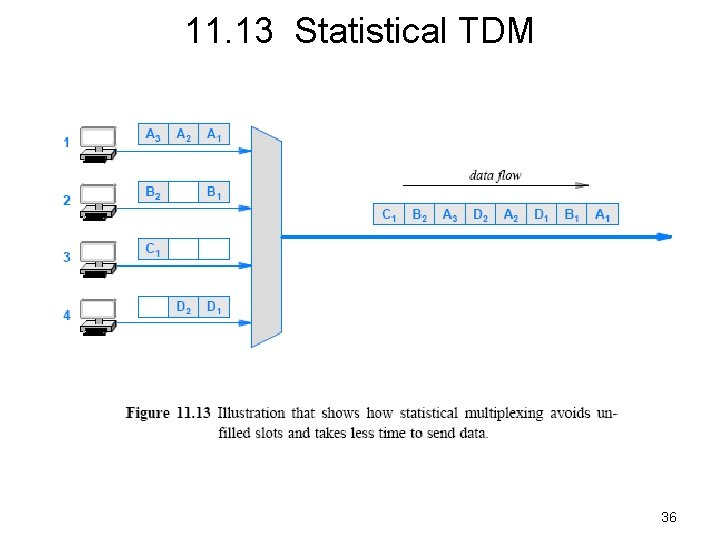11. 13 Statistical TDM 36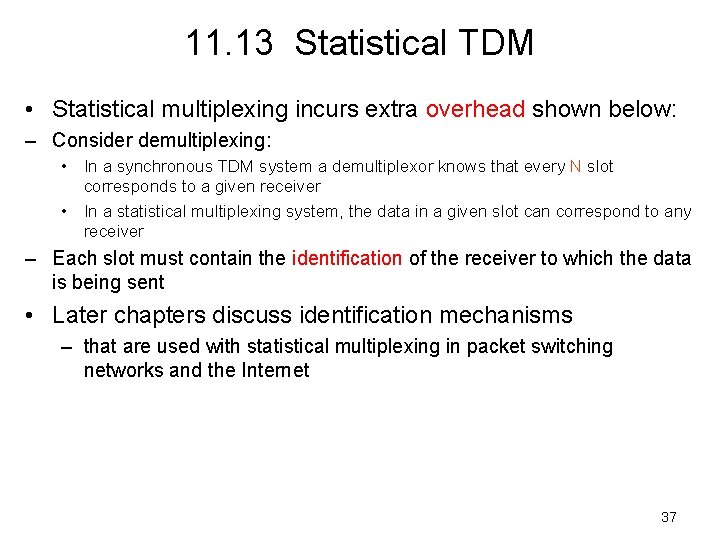11. 13 Statistical TDM • Statistical multiplexing incurs extra overhead shown below: – Consider demultiplexing: • In a synchronous TDM system a demultiplexor knows that every N slot corresponds to a given receiver • In a statistical multiplexing system, the data in a given slot can correspond to any receiver – Each slot must contain the identification of the receiver to which the data is being sent • Later chapters discuss identification mechanisms – that are used with statistical multiplexing in packet switching networks and the Internet 37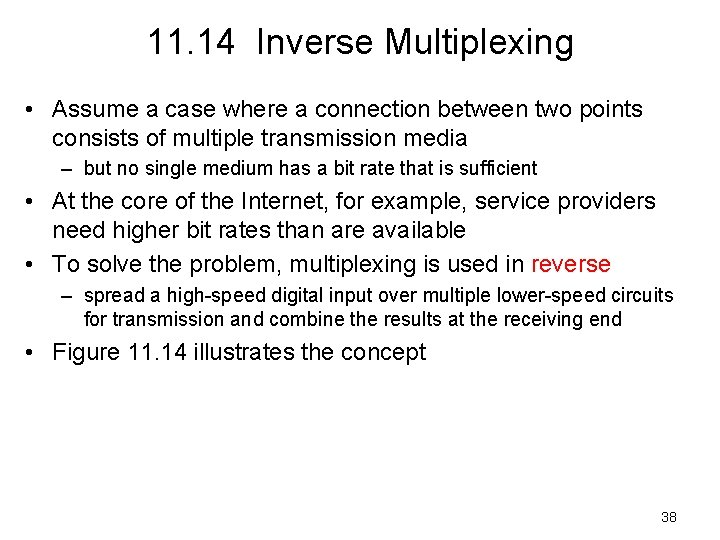11. 14 Inverse Multiplexing • Assume a case where a connection between two points consists of multiple transmission media – but no single medium has a bit rate that is sufficient • At the core of the Internet, for example, service providers need higher bit rates than are available • To solve the problem, multiplexing is used in reverse – spread a high-speed digital input over multiple lower-speed circuits for transmission and combine the results at the receiving end • Figure 11. 14 illustrates the concept 38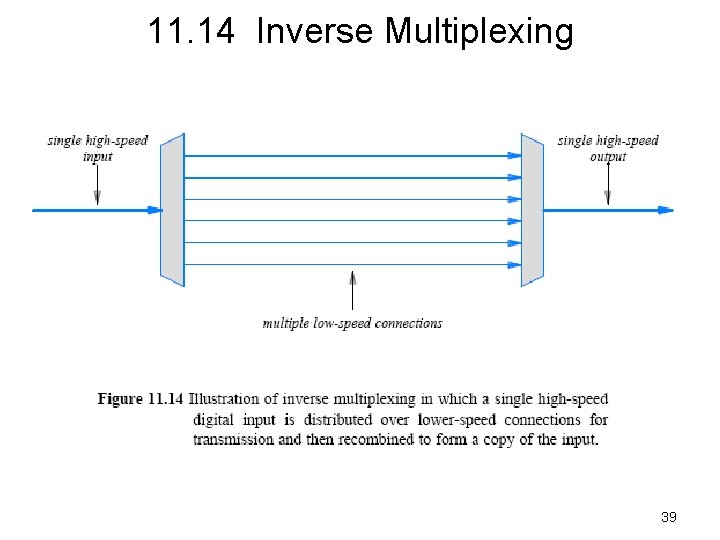11. 14 Inverse Multiplexing 3911. 14 Inverse Multiplexing • An inverse multiplexor cannot be constructed by connecting the pieces of a conventional multiplexor backward – hardware must be designed so that the sender and receiver agree on how data arriving from the input will be distributed over the lowerspeed connections – to insure that all data is delivered in the same order as it arrived, the system must be engineered to handle cases • where one or more of the lower-speed connections has longer latency than others • Despite its complexity, inverse multiplexing is widely used in the Internet 40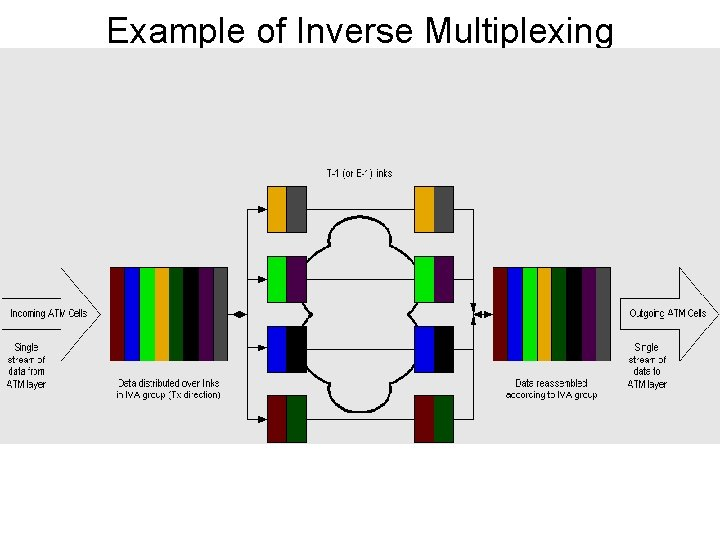Example of Inverse Multiplexing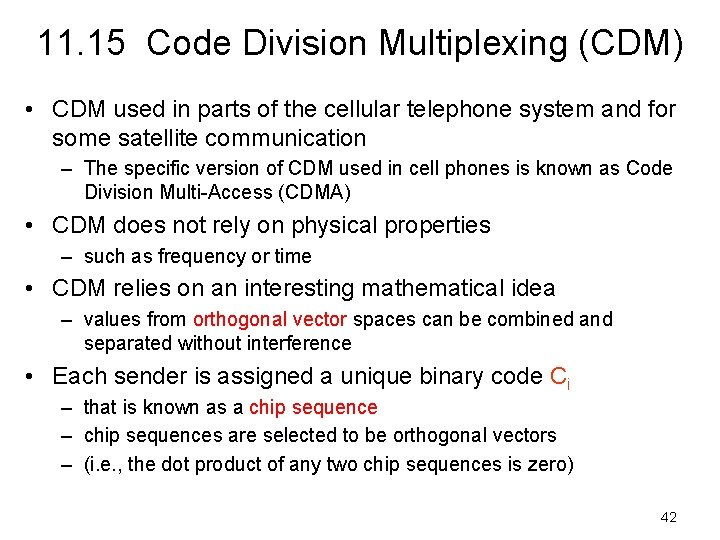11. 15 Code Division Multiplexing (CDM) • CDM used in parts of the cellular telephone system and for some satellite communication – The specific version of CDM used in cell phones is known as Code Division Multi-Access (CDMA) • CDM does not rely on physical properties – such as frequency or time • CDM relies on an interesting mathematical idea – values from orthogonal vector spaces can be combined and separated without interference • Each sender is assigned a unique binary code Ci – that is known as a chip sequence – chip sequences are selected to be orthogonal vectors – (i. e. , the dot product of any two chip sequences is zero) 4211. 15 Code Division Multiplexing • At any point in time, each sender has a value to transmit, Vi – The senders each multiply Ci x Vi and transmit the results • The senders transmit at the same time – and the values are added together • To extract value Vi, a receiver multiplies the sum by Ci • Consider an example – to keep the example easy to understand, use a chip sequence that is only two bits long and data values that are four bits long – think of the chip sequence as a vector • Figure 11. 15 lists the values 43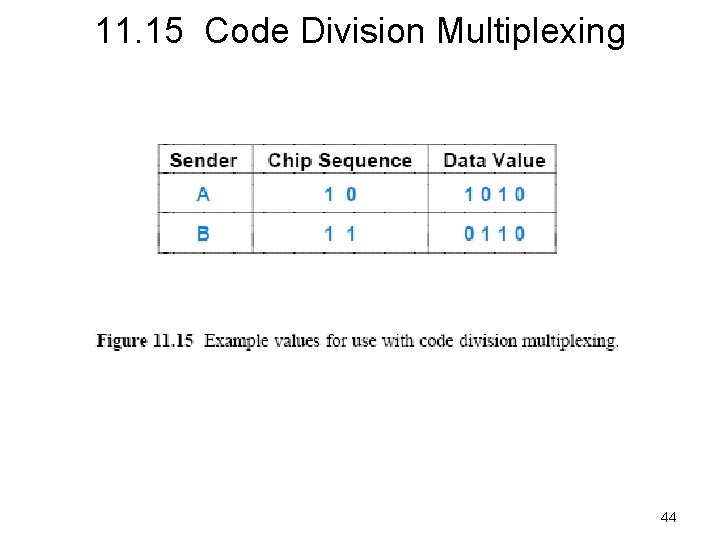11. 15 Code Division Multiplexing 4411. 15 Code Division Multiplexing • The first step consists of converting the binary values into vectors that use -1 to represent 0: • If we think of the resulting values as a sequence of signal strengths to be transmitted at the same time – the resulting signal will be the sum of the two signals 45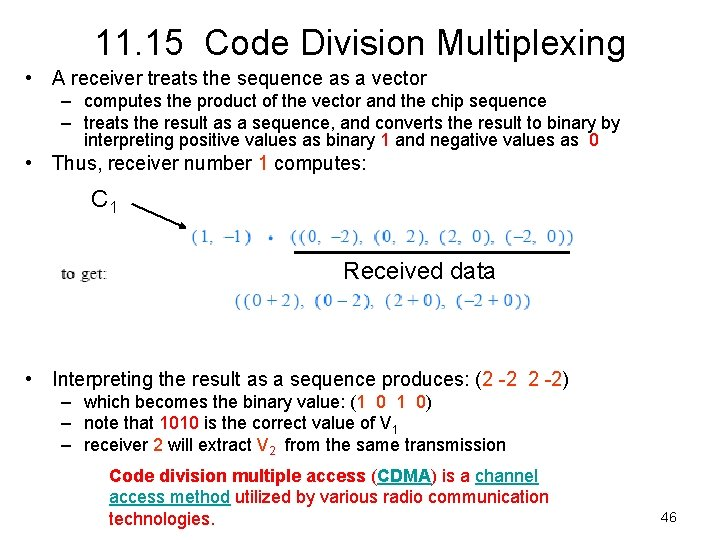11. 15 Code Division Multiplexing • A receiver treats the sequence as a vector – computes the product of the vector and the chip sequence – treats the result as a sequence, and converts the result to binary by interpreting positive values as binary 1 and negative values as 0 • Thus, receiver number 1 computes: C 1 Received data • Interpreting the result as a sequence produces: (2 -2) – which becomes the binary value: (1 0 1 0) – note that 1010 is the correct value of V 1 – receiver 2 will extract V 2 from the same transmission Code division multiple access (CDMA) is a channel access method utilized by various radio communication technologies. 46